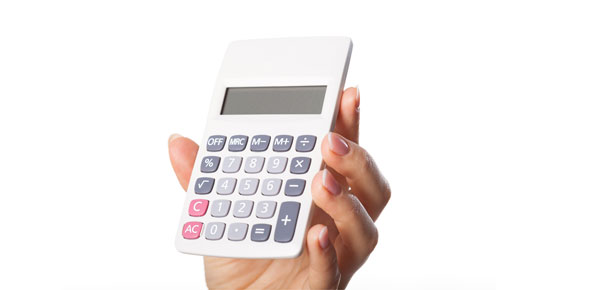# Quiz Calculate

10 Questions | Total Attempts: 46Settings.

• 1.
Round off 10 021 correct to one significant figure?
• A.

1

• B.

2

• C.

10 000

• D.

20 000

• 2.
Round off 0.0012429 correct to two significant figures?
• A.

0.00012

• B.

0.0012

• C.

0.00120

• D.

0.0013

• 3.
Express  as a single number.
• A.

7448

• B.

744.8

• C.

74.48

• D.

7.448

• 4.
A rectangular floor of a school hall has a length of 18 m and a width of 34 m. Square tiles with length 12 cm is used to cover the floor. Calculate the number of tiles required to cover the floor completely.
• A.
• B.
• C.
• D.
• 5.
• A.
• B.
• C.
• D.
• 6.
State whether this statement is true or false.
• A.

True

• B.

False

• 7.
State whether this statement is true or false. The elements of set  are multiples of 5 or the elements of set  are perfect squares.
• A.

True

• B.

False

• 8.
Write down Premise 2 to complete the following argument: Premise 1   :  If b is a perfect square, then  is an integer Premise 2   :  ---------------------------------------------------------------- Conclusion :   is an integer
• 9.
Write down the converse of the following implication: If k is a multiple of 25, then k is multiple of 5
• 10.
Complete the following statements with the quantifiers "all" or "some" so that it will become a true statement. -------------------  perfect squares are odd numbers.
Related Topics